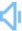Directly to word page Vague search(google)

## Percentage in a sentence

Sentence count:246+10 Only show simple sentencesPosted:2016-07-16Updated:2020-07-24
Synonym: partrateSimilar words: Meaning: [pər'sentɪdʒ /pə-]n. 1. a proportion multiplied by 100 2. assets belonging to or due to or contributed by an individual person or group.Random good picture Not show
1. The figure is expressed as a percentage.
2. The percentage of girls in engineering has increased substantially.
3. Whisky contains a large percentage of alcohol.
4. The area has a high percentage of unemployed men.
4. Sentencedict.com is a online sentence dictionary, on which you can find nice sentences for a large number of words.
5. What percentage of the students are admitted to college?
6. What percentage of the students were absent?
7. The percentage has increased by 15 to 44.
8. Television attracts a large percentage of the people.
9. A surprising percentage of the population are illiterate.
10. Unfortunately belts are worn only by a small percentage of drivers and passengers.
11. A small percentage reduction in the cost of materials would mean a significant increase in profit.
12. The percentage of school leavers that go to university is about five per cent.
13. She gets a percentage for every record sold.
14. What percentage of eligible voters cast their ballots?
15. The artist's agent receives commission on a percentage basis.
16. Crime figures showed significant percentage increases.
17. What percentage of the population is / are overweight?
18. A large percentage of the rural population was illiterate.
19. A high percentage of married women have part-time jobs.
20. He gets a percentage for every car sold.
21. Tax is paid as a percentage of total income.
22. The percentage of school-leavers is shown on the chart.
23. What is the percentage of nitrogen in air?
24. The numbers are relatively low in percentage terms.
25. There's no percentage in working such long hours.
26. Interest rates fell by six percentage points .
27. Interest rates fell by one percentage point.
28. What percentage of the adult male population is unemployed?
29. There's no percentage in worrying.
30. Interest rates have risen by two percentage points.
Total 246, 30 Per page  1/9  «first  next  last»  goto